# Precalculus : Special Functions

## Example Questions

### Example Question #1 : Piecewise Functions

Let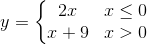What doesequal when?Explanation:

Because 3>0 we plug the x value into the bottom equation.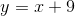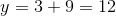### Example Question #2 : Piecewise Functions

Let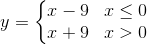What doesequal when?Explanation:

Because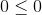we use the first equation.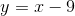Therefore, plugging in x=0 into the above equation we get the following,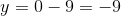.

### Example Question #3 : Piecewise Functions

Determine the value of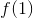if the function is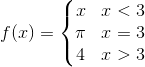Explanation:

In order to determine the value ofof the function we set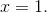The value comes from the function in the first row of the piecewise function, and as such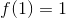### Example Question #21 : Functions

Determine the value ofif the function is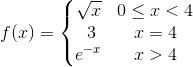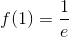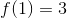Explanation:

In order to determine the value ofof the function we setThe value comes from the function in the first row of the piecewise function, and as such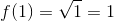### Example Question #1 : Piecewise Functions

For the functiondefined below, what is the value ofwhen?8

0

-2

7

3

8

Explanation:

Evaluate the function for. Based on the domains of the three given expressions, you would use, sinceis greater than or equal to.### Example Question #1 : Greatest Integer Functions

Ifis the greatest integer function, what is the value of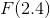Explanation:

The greatest integer function takes an input and produces the greatest integer less than the input. Thus, the output is always smaller than the input and is an integer itself. Since our input was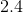, we are looking for an integer less than this, which must besince any smaller integer would by definition not be "greatest".

### Example Question #1 : Special Functions

Which of the following is a point on the following function?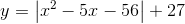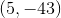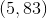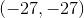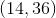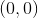Explanation:

One way to approach this problem would be to plug in each answer and see what works. However, I would be a little more strategic and eliminate any options that don't make sense.

Our y value will never be negative, so eliminate any options with a negative y-value.

Try (0,0) really quick, since it's really easy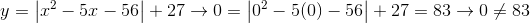The only point that makes sense is (5,83), therefore it is the correct answer### Example Question #1 : Special Functions

Evaluate: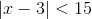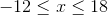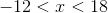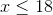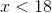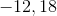Explanation:

Cancel the absolute value sign by separating the functioninto its positive and negative counterparts.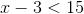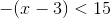Evaluate the first scenario.Evaluate the second scenario.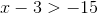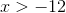### Example Question #3 : Absolute Value Functions

If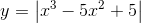, then what is the value ofwhen?

20

-13

7

-7

13

13

Explanation:

We evaluate for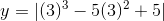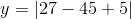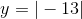Since the absolute value of any number represents its magnitude fromand is therefore always positive, the final answer would be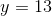### All Precalculus Resources